# polarscatter

Scatter chart in polar coordinates

•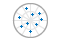## Syntax

``polarscatter(theta,rho)``
``polarscatter(theta,rho,sz)``
``polarscatter(theta,rho,sz,c)``
``polarscatter(___,mkr)``
``polarscatter(___,'filled')``
``polarscatter(tbl,thetavar,rhovar)``
``polarscatter(tbl,thetavar,rhovar,'filled')``
``polarscatter(pax,___)``
``polarscatter(___,Name,Value)``
``ps = polarscatter(___)``

## Description

### Vector and Matrix Data

example

````polarscatter(theta,rho)` plots `theta` versus `rho` and displays a circular marker at each data point. `theta` and `rho` must be vectors of the same length. You must specify `theta` in radians. To plot one set of points, specify `theta` and `rho` as vectors of equal length.To plot multiple sets of points within the same polar axes, specify at least one of `theta` or `rho` as a matrix. ```
````polarscatter(theta,rho,sz)` sets the marker sizes, where `sz` specifies the area of each marker in points squared. To draw all the markers with the same size, specify `sz` as a scalar. To draw the markers with different sizes, as a vector or a matrix.```

example

````polarscatter(theta,rho,sz,c)` specifies the marker colors. You can specify one color for all the markers, or you can vary the color. For example, you can plot all red circles by specifying `c` as `'red'`.```
````polarscatter(___,mkr)` sets the marker symbol. For example, `'+'` displays cross markers. Specify the marker symbol after any of the input argument combinations in the previous syntaxes.```

example

````polarscatter(___,'filled')` fills the marker interiors.```

### Table Data

example

````polarscatter(tbl,thetavar,rhovar)` plots the variables `thetavar` and `rhovar` from the table `tbl`. To plot one data set, specify one variable for `thetavar` and one variable for `rhovar`. To plot multiple data sets, specify multiple variables for `thetavar`, `rhovar`, or both. If both arguments specify multiple variables, they must specify the same number of variables. (Since R2021b)```

example

````polarscatter(tbl,thetavar,rhovar,'filled')` plots the specified variables from the table with filled circles. (Since R2021b)```

````polarscatter(pax,___)` plots into the polar axes specified by `pax` instead of into the current axes.```
````polarscatter(___,Name,Value)` modifies the appearance of the scatter plot using one or more name-value pair arguments. For example, you can use semi-transparent markers by specifying `'FaceAlpha'` and a scalar value between `0` and `1`.```

example

````ps = polarscatter(___)` returns the `Scatter` object or an array of `Scatter` objects.. Use `ps` to modify the appearance of the `Scatter` object after it is created. For a list of properties, see Scatter Properties.```

## Examples

collapse all

Create a scatter chart in polar coordinates.

```th = pi/4:pi/4:2*pi; r = [19 6 12 18 16 11 15 15]; polarscatter(th,r)```Create a scatter chart that uses filled markers by specifying the optional input argument, `'filled'`. Set the marker size to 75 points squared.

```th = linspace(0,2*pi,20); r = rand(1,20); sz = 75; polarscatter(th,r,sz,'filled')```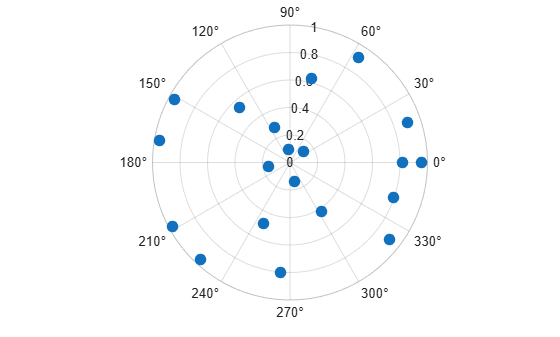Create a scatter chart with markers of varying sizes and colors. Specify the optional size and color input arguments as vectors. Use unique values in the color vector to specify the different colors you want. The values map to colors in the colormap.

```th = pi/4:pi/4:2*pi; r = [19 6 12 18 16 11 15 15]; sz = 100*[6 15 20 3 15 3 6 40]; c = [1 2 2 2 1 1 2 1]; polarscatter(th,r,sz,c,'filled','MarkerFaceAlpha',.5)```Create data where the angle values are in degrees. Since `polarscatter` requires angle values in radians, convert the values to radians before plotting using `deg2rad`.

```th = linspace(0,360,50); r = 0.005*th/10; th_radians = deg2rad(th); polarscatter(th_radians,r)```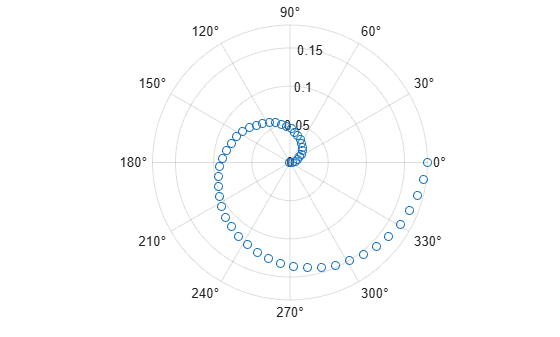Combine two scatter charts in the same polar axes using the `hold` command. Add a legend with a description of each chart.

```th = pi/6:pi/6:2*pi; r1 = rand(12,1); polarscatter(th,r1,'filled') hold on r2 = rand(12,1); polarscatter(th,r2,'filled') hold off legend('Series A','Series B')```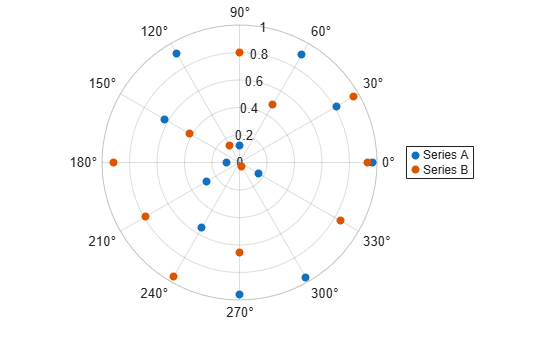Create a scatter chart and assign the scatter object to the variable `ps`.

```th = pi/6:pi/6:2*pi; r = rand(12,1); ps = polarscatter(th,r,'filled')```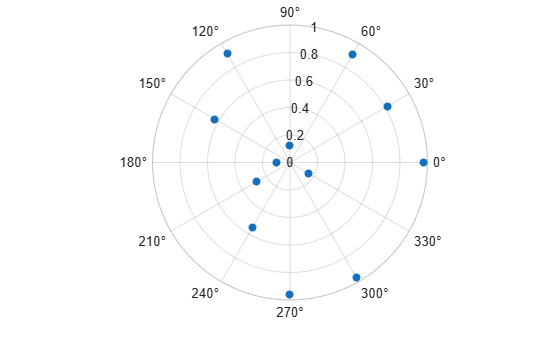```ps = Scatter with properties: ThetaData: [0.5236 1.0472 1.5708 2.0944 2.6180 3.1416 3.6652 ... ] RData: [0.8147 0.9058 0.1270 0.9134 0.6324 0.0975 0.2785 ... ] ZData: [1x0 double] SizeData: 36 CData: [0 0.4470 0.7410] Marker: 'o' MarkerEdgeColor: 'none' MarkerFaceColor: 'flat' LineWidth: 0.5000 Show all properties ```

Use `ps` to modify properties of the scatter object after it is created.

```ps.Marker = 'square'; ps.SizeData = 200; ps.MarkerFaceColor = 'red'; ps.MarkerFaceAlpha = .5;```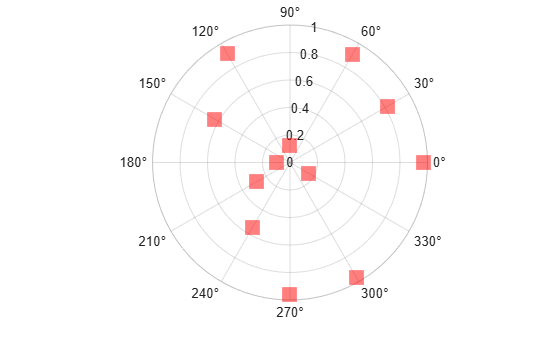Since R2021b

A convenient way to plot data from a table is to pass the table to the `polarscatter` function and specify the variables you want to plot. For example, create a table with three variables, and plot the `'Th'` and `'R1'` variables.

```% Create a table of random numbers Th = linspace(0,2*pi,50)'; R1 = randi([0 10],50,1); R2 = randi([20 30],50,1); tbl = table(Th,R1,R2); % Create polar scatter chart polarscatter(tbl,'Th','R1')```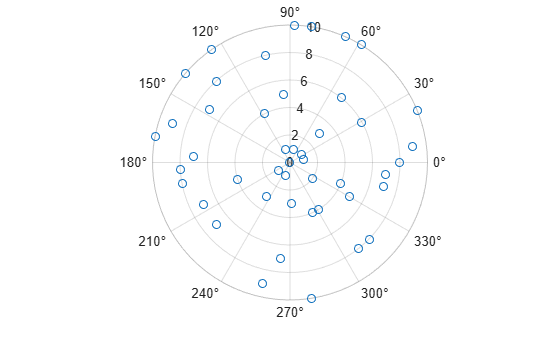You can also plot multiple variables at the same time. For example, plot two sets of radius values by specifying the `rvar` argument as the cell array `{'R1','R2'}`. Then add a legend. The legend labels match the variable names.

```polarscatter(tbl,'Th',{'R1','R2'}) legend```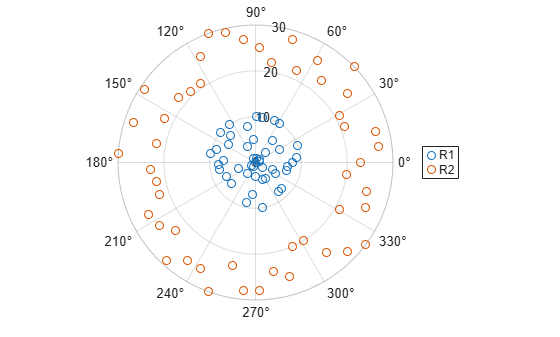Since R2021b

One way to plot data from a table and customize the colors and marker sizes is to set the `ColorVariable` and `SizeData` properties. You can set these properties as name-value arguments when you call the `polarscatter` function, or you can set them on the `Scatter` object later.

For example, create a table with three variables of random numbers, and plot the `Th` `and` `R` variables with filled markers. Vary the marker colors by specifying the `ColorVariable` name-value argument. Return the `Scatter` object as `s`, so you can set other properties later.

```Th = linspace(0,2*pi,50)'; R = randi([0 10],50,1); Colors = rand(50,1); tbl = table(Th,R,Colors); s = polarscatter(tbl,'Th','R','filled','ColorVariable','Colors');```Change the marker sizes to 100 points by setting the `SizeData` property.

`s.SizeData = 100;`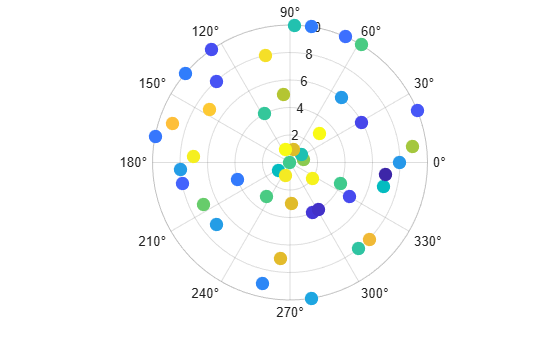## Input Arguments

collapse all

Theta values, specified as a scalar, vector, or matrix. The `ThetaData` property of the scatter object stores the theta values.

The size and shape of `theta` depends on the shape of your data. This table describes the most common situations.

Type of PlotHow to Specify Coordinates
Single point

Specify `theta` and `rho` as scalars. For example:

`polarscatter(pi/2,0.5)`

One set of points

Specify `theta` and `rho` as any combination of row or column vectors of the same length. For example:

`polarscatter([0 pi/4 pi/2],[1; 2; 3])`

Multiple sets of points that are different colors

If all the sets share the same theta or rho values, specify the shared coordinates as a vector and the other coordinates as a matrix. The length of the vector must match one of the dimensions of the matrix. For example:

`polarscatter([0 pi/4 pi/2],[4 5 6; 7 8 9])`
If the matrix is square, `polarscatter` plots a separate set of points for each column in the matrix.

Alternatively, specify `theta` and `rho` as matrices of equal size. In this case, `polarscatter` plots each column of `theta` against the corresponding column of `rho`. For example:

`polarscatter([0 pi/6 pi/2; pi/8 pi/4 pi],[1 2 3; 4 5 6])`

To convert degrees to radians, use `deg2rad`.

Data Types: `single` | `double` | `int8` | `int16` | `int32` | `int64` | `uint8` | `uint16` | `uint32` | `uint64`

Rho values, specified as a scalar, vector, or matrix. The `RData` property of the scatter object stores the rho values.

The size and shape of `rho` depends on the shape of your data. This table describes the most common situations.

Type of PlotHow to Specify Coordinates
Single point

Specify `theta` and `rho` as scalars. For example:

`polarscatter(pi/2,0.5)`

One set of points

Specify `theta` and `rho` as any combination of row or column vectors of the same length. For example:

`polarscatter([0 pi/4 pi/2],[1; 2; 3])`

Multiple sets of points that are different colors

If all the sets share the same theta or rho values, specify the shared coordinates as a vector and the other coordinates as a matrix. The length of the vector must match one of the dimensions of the matrix. For example:

`polarscatter([0 pi/4 pi/2],[4 5 6; 7 8 9])`
If the matrix is square, `polarscatter` plots a separate set of points for each column in the matrix.

Alternatively, specify `theta` and `rho` as matrices of equal size. In this case, `polarscatter` plots each column of `theta` against the corresponding column of `rho`. For example:

`polarscatter([0 pi/6 pi/2; pi/8 pi/4 pi],[1 2 3; 4 5 6])`

Data Types: `single` | `double` | `int8` | `int16` | `int32` | `int64` | `uint8` | `uint16` | `uint32` | `uint64`

Marker size, specified as a numeric scalar, vector, matrix, or empty array (`[]`). The size controls the area of each marker in points squared. An empty array specifies the default size of 36 points. The way you specify the size depends on how you specify `theta` and `rho`, and how you want the plot to look. This table describes the most common situations.

Desired Marker Sizes`theta` and `rho` `sz`Example

Same size for all points

Any valid combination of vectors or matrices described for `theta` and `rho`.

Scalar

Specify `theta` as a vector, `rho` as a matrix, and `sz` as a scalar.

```th = [0 pi/6 pi/2]; r = [1 2; 2 4; 5 6]; polarscatter(th,r,100)```

Different size for each point

Vectors of the same length

• A vector with the same length as `theta` and `rho`.

• A matrix with at least one dimension that matches the lengths of `theta` and `rho`. Specifying a matrix is useful for displaying multiple markers with different sizes at each (th,r) location.

Specify `theta`, `rho`, and `sz` as vectors.

```th = [0 pi/6 pi/2]; r = [1; 2; 3]; sz = [50 500 100]; polarscatter(th,r,sz)```

Specify `theta` and `rho` as vectors and `sz` as a matrix.

```th = [0 pi/6 pi/2]; r = [1; 2; 3]; sz = [50 500 100; 300 1000 200]; polarscatter(th,r,sz)```

Different size for each point

At least one of `x` or `y` is a matrix for plotting multiple data sets

• A vector with the same number of elements as there are points in each data set.

• A matrix that has the same size as the `theta` or `rho` matrix.

Specify `theta` as a vector, `rho` as a matrix, and `sz` as vector.

```th = [0 pi/6 pi/2]; r = [1 2; 2 4; 5 6]; sz = [50 500 1000]; polarscatter(th,r,sz)```

Specify `theta` as a vector, `rho` as a matrix, and `sz` as a matrix the same size as `rho`.

```th = [0 pi/6 pi/2]; r = [1 2; 2 4; 5 6]; sz = [50 500; 1000 2000; 100 300]; polarscatter(th,r,sz)```

Data Types: `single` | `double` | `int8` | `int16` | `int32` | `int64` | `uint8` | `uint16` | `uint32` | `uint64`

Marker color, specified as a color name, RGB triplet, matrix of RGB triplets, or a vector of colormap indices.

• Color name — A color name such as `'red'`, or a short name such as `'r'`.

• RGB triplet — A three-element row vector whose elements specify the intensities of the red, green, and blue components of the color. The intensities must be in the range `[0,1]`; for example, ```[0.4 0.6 0.7]```. RGB triplets are useful for creating custom colors.

• Matrix of RGB triplets — A three-column matrix in which each row is an RGB triplet.

• Vector of colormap indices — A vector of numeric values that is the same length as the `theta` and `rho` vectors.

The way you specify the color depends on the desired color scheme and whether you are plotting one set of coordinates or multiple sets of coordinates. This table describes the most common situations.

Color SchemeHow to Specify the ColorExample

Use one color for all the points.

Specify a color name or a short name from the table below, or specify one RGB triplet.

Plot one set of points, and specify the color as `'red'`.

```th = [0 pi/6 pi/2]; r = [1 2 3]; c = 'red'; polarscatter(th,r,[],c)```

Plot two sets of points, and specify the color as red using an RGB triplet.

```th = [0 pi/6 pi/2]; r = [1 2; 2 4; 5 6]; c = [1 0 0]; polarscatter(th,r,[],c)```

Assign different colors to each point using a colormap.

Specify a row or column vector of numbers. The numbers index into the current colormap array. The smallest value maps to the first row in the colormap, and the largest value maps to the last row. The intermediate values map linearly to the intermediate rows.

If your plot has three points, specify a column vector to ensure the values are interpreted as colormap indices.

You can use this method only when `theta`, `rho`, and `sz` are all vectors.

Create a vector `c` that specifies four colormap indices. Plot four points using the colors from the current colormap. Then, change the colormap to `winter`.

```th = [0 pi/6 pi/2 2*pi/3]; r = [1 2 3 4]; c = [1 2 3 4]; polarscatter(th,r,[],c) colormap(gca,'winter')```

Create a custom color for each point.

Specify an m-by-3 matrix of RGB triplets, where m is the number of points in the plot.

You can use this method only when `theta`, `rho`, and `sz` are all vectors.

Create a matrix `c` that specifies the RGB triplets of four colors. Then create plot four points using those colors.

```th = [0 pi/6 pi/2 2*pi/3]; r = [1 2 3 4]; c = [1 0 0; 0 1 0; 0 0 1; 0 0 0]; polarscatter(th,r,[],c)```

Create a different color for each data set.

Specify an n-by-3 matrix of RGB triplets, where n is the number of data sets.

You can use this method only when at least one of `theta`, `rho`, or `sz` is a matrix.

Create a matrix `c` that contains two RGB triplets. Then plot two data sets using those colors.

```th = [0 pi/6 pi/2]; r = [1 2; 2 4; 5 6]; c = [1 0 0; 0 0 1]; polarscatter(th,r,[],c)```

#### Color Names and RGB Triplets for Common Colors

Color NameShort NameRGB TripletHexadecimal Color CodeAppearance
`'red'``'r'``[1 0 0]``'#FF0000'``'green'``'g'``[0 1 0]``'#00FF00'``'blue'``'b'``[0 0 1]``'#0000FF'``'cyan'` `'c'``[0 1 1]``'#00FFFF'``'magenta'``'m'``[1 0 1]``'#FF00FF'``'yellow'``'y'``[1 1 0]``'#FFFF00'``'black'``'k'``[0 0 0]``'#000000'``'white'``'w'``[1 1 1]``'#FFFFFF'`Here are the RGB triplets and hexadecimal color codes for the default colors MATLAB® uses in many types of plots.

`[0 0.4470 0.7410]``'#0072BD'``[0.8500 0.3250 0.0980]``'#D95319'``[0.9290 0.6940 0.1250]``'#EDB120'``[0.4940 0.1840 0.5560]``'#7E2F8E'``[0.4660 0.6740 0.1880]``'#77AC30'``[0.3010 0.7450 0.9330]``'#4DBEEE'``[0.6350 0.0780 0.1840]``'#A2142F'`Data Types: `single` | `double` | `int8` | `int16` | `int32` | `int64` | `uint8` | `uint16` | `uint32` | `uint64` | `char` | `string`

Marker symbol, specified as one of the marker symbols listed in this table.

MarkerDescriptionResulting Marker
`'o'`Circle`'+'`Plus sign`'*'`Asterisk`'.'`Point`'x'`Cross`'_'`Horizontal line`'|'`Vertical line`'s'`Square`'d'`Diamond`'^'`Upward-pointing triangle`'v'`Downward-pointing triangle`'>'`Right-pointing triangle`'<'`Left-pointing triangle`'p'`Pentagram`'h'`HexagramThe `Marker` property of the scatter object stores the marker symbol.

Source table containing the data to plot. Specify this argument as a table or a timetable.

Table variables containing the theta values, specified as one or more table variable indices.

#### Specifying Table Indices

Use any of the following indexing schemes to specify the desired variable or variables.

Indexing SchemeExamples

Variable names:

• A character vector or a string scalar.

• A cell array or string vector.

• `'A'` or `"A"` — A variable called `A`

• `{'A','B'}` or `["A","B"]` — Two variables called `A` and `B`

Variable numbers:

• An index number that refers to the location of a variable in the table.

• A vector of numbers.

• `3` — The third variable from the table

• `[2 3]` — The second and third variables from the table

Logical vector:

• An `n`-element vector logical vector, where `logical 1` (`true`) values indicate the desired variables.

• Optionally, omit the trailing `false` values after the last `true` value.

• `[false false true false]` — The third variable from an `m`-by-`4` table

• `[false false true]` — The third variable from any size table

• `[false true true]` — The second and third variables from any size table

Variable type:

• `vartype('categorical')` — All the variables containing categorical values

The table variables you specify can contain any numeric data type.

To plot one data set, specify one variable for `thetavar` and one variable for `rhovar`. For example, create a table with three variables. Plot the `Th` and `R1` variables.

```% Create a table of random numbers Th = linspace(0,2*pi,10)'; R1 = randi([0 10],10,1); R2 = randi([20 30],10,1); tbl = table(Th,R1,R2); % Create polar scatter chart polarscatter(tbl,'Th','R1')```

To plot multiple data sets together, specify multiple variables for `thetavar`, `rhovar`, or both. If you specify multiple variables for both arguments, the number of variables for each argument must be the same.

For example, plot the `Th` variable on the theta-axis and the `R1` and `R2` variables on the r-axis.

`polarscatter(tbl,'Th',{'R1','R2'})`

You can also use different indexing schemes for the table variables. For example, specify `thetavar` as a variable name and `rhovar` as an index number.

`polarscatter(tbl,'Th',2)`

Table variables containing the rho values, specified as one or more table variable indices.

#### Specifying Table Indices

Use any of the following indexing schemes to specify the desired variable or variables.

Indexing SchemeExamples

Variable names:

• A character vector or a string scalar.

• A cell array or string vector.

• `'A'` or `"A"` — A variable called `A`

• `{'A','B'}` or `["A","B"]` — Two variables called `A` and `B`

Variable numbers:

• An index number that refers to the location of a variable in the table.

• A vector of numbers.

• `3` — The third variable from the table

• `[2 3]` — The second and third variables from the table

Logical vector:

• An `n`-element vector logical vector, where `logical 1` (`true`) values indicate the desired variables.

• Optionally, omit the trailing `false` values after the last `true` value.

• `[false false true false]` — The third variable from an `m`-by-`4` table

• `[false false true]` — The third variable from any size table

• `[false true true]` — The second and third variables from any size table

Variable type:

• `vartype('categorical')` — All the variables containing categorical values

The table variables you specify can contain any numeric data type.

To plot one data set, specify one variable for `thetavar` and one variable for `rhovar`. For example, create a table with three variables. Plot the `Th` and `R1` variables.

```% Create a table of random numbers Th = linspace(0,2*pi,10)'; R1 = randi([0 10],10,1); R2 = randi([20 30],10,1); tbl = table(Th,R1,R2); % Create polar scatter chart polarscatter(tbl,'Th','R1')```

To plot multiple data sets together, specify multiple variables for `thetavar`, `rhovar`, or both. If you specify multiple variables for both arguments, the number of variables for each argument must be the same.

For example, plot the `Th` variable on the theta-axis and the `R1` and `R2` variables on the r-axis.

`polarscatter(tbl,'Th',{'R1','R2'})`

You can also use different indexing schemes for the table variables. For example, specify `thetavar` as a variable name and `rhovar` as an index number.

`polarscatter(tbl,'Th',2)`

`PolarAxes` object. If you do not specify the polar axes, then `polarscatter` uses the current axes. `polarscatter` does not support plotting into Cartesian axes.

### Name-Value Arguments

Specify optional comma-separated pairs of `Name,Value` arguments. `Name` is the argument name and `Value` is the corresponding value. `Name` must appear inside quotes. You can specify several name and value pair arguments in any order as `Name1,Value1,...,NameN,ValueN`.

Example: `polarscatter(th,r,'filled','MarkerFaceAlpha',.5)` creates filled, semi-transparent markers.

The scatter object properties listed here are only a subset. For a complete list, see Scatter Properties.

Marker face transparency, specified as a scalar in the range `[0,1]`. A value of 1 is opaque and 0 is transparent. Values between 0 and 1 are semi-transparent.

Marker outline color, specified `'flat'`, an RGB triplet, a hexadecimal color code, a color name, or a short name. The default value of `'flat'` uses colors from the `CData` property.

For a custom color, specify an RGB triplet or a hexadecimal color code.

• An RGB triplet is a three-element row vector whose elements specify the intensities of the red, green, and blue components of the color. The intensities must be in the range `[0,1]`; for example, ```[0.4 0.6 0.7]```.

• A hexadecimal color code is a character vector or a string scalar that starts with a hash symbol (`#`) followed by three or six hexadecimal digits, which can range from `0` to `F`. The values are not case sensitive. Thus, the color codes `'#FF8800'`, `'#ff8800'`, `'#F80'`, and `'#f80'` are equivalent.

Alternatively, you can specify some common colors by name. This table lists the named color options, the equivalent RGB triplets, and hexadecimal color codes.

Color NameShort NameRGB TripletHexadecimal Color CodeAppearance
`'red'``'r'``[1 0 0]``'#FF0000'``'green'``'g'``[0 1 0]``'#00FF00'``'blue'``'b'``[0 0 1]``'#0000FF'``'cyan'` `'c'``[0 1 1]``'#00FFFF'``'magenta'``'m'``[1 0 1]``'#FF00FF'``'yellow'``'y'``[1 1 0]``'#FFFF00'``'black'``'k'``[0 0 0]``'#000000'``'white'``'w'``[1 1 1]``'#FFFFFF'``'none'`Not applicableNot applicableNot applicableNo color

Here are the RGB triplets and hexadecimal color codes for the default colors MATLAB uses in many types of plots.

`[0 0.4470 0.7410]``'#0072BD'``[0.8500 0.3250 0.0980]``'#D95319'``[0.9290 0.6940 0.1250]``'#EDB120'``[0.4940 0.1840 0.5560]``'#7E2F8E'``[0.4660 0.6740 0.1880]``'#77AC30'``[0.3010 0.7450 0.9330]``'#4DBEEE'``[0.6350 0.0780 0.1840]``'#A2142F'`Example: `[0.5 0.5 0.5]`

Example: `'blue'`

Example: `'#D2F9A7'`

Marker fill color, specified as `'flat'`, `'auto'`, an RGB triplet, a hexadecimal color code, a color name, or a short name. The `'flat'` option uses the `CData` values. The `'auto'` option uses the same color as the `Color` property for the axes.

For a custom color, specify an RGB triplet or a hexadecimal color code.

• An RGB triplet is a three-element row vector whose elements specify the intensities of the red, green, and blue components of the color. The intensities must be in the range `[0,1]`; for example, ```[0.4 0.6 0.7]```.

• A hexadecimal color code is a character vector or a string scalar that starts with a hash symbol (`#`) followed by three or six hexadecimal digits, which can range from `0` to `F`. The values are not case sensitive. Thus, the color codes `'#FF8800'`, `'#ff8800'`, `'#F80'`, and `'#f80'` are equivalent.

Alternatively, you can specify some common colors by name. This table lists the named color options, the equivalent RGB triplets, and hexadecimal color codes.

Color NameShort NameRGB TripletHexadecimal Color CodeAppearance
`'red'``'r'``[1 0 0]``'#FF0000'``'green'``'g'``[0 1 0]``'#00FF00'``'blue'``'b'``[0 0 1]``'#0000FF'``'cyan'` `'c'``[0 1 1]``'#00FFFF'``'magenta'``'m'``[1 0 1]``'#FF00FF'``'yellow'``'y'``[1 1 0]``'#FFFF00'``'black'``'k'``[0 0 0]``'#000000'``'white'``'w'``[1 1 1]``'#FFFFFF'``'none'`Not applicableNot applicableNot applicableNo color

Here are the RGB triplets and hexadecimal color codes for the default colors MATLAB uses in many types of plots.

`[0 0.4470 0.7410]``'#0072BD'``[0.8500 0.3250 0.0980]``'#D95319'``[0.9290 0.6940 0.1250]``'#EDB120'``[0.4940 0.1840 0.5560]``'#7E2F8E'``[0.4660 0.6740 0.1880]``'#77AC30'``[0.3010 0.7450 0.9330]``'#4DBEEE'``[0.6350 0.0780 0.1840]``'#A2142F'`Example: `[0.3 0.2 0.1]`

Example: `'green'`

Example: `'#D2F9A7'`

Width of marker edge, specified as a positive value in point units.

Example: `0.75`

Table variable containing the color data, specified as a variable index into the source table.

#### Specifying the Table Index

Use any of the following indexing schemes to specify the desired variable.

Indexing SchemeExamples

Variable name:

A character vector or a string scalar.

• `'A'` or `"A"` — A variable called `A`

Variable number:

An index number that refers to the location of a variable in the table.

• `3` — The third variable from the table

Logical vector:

• An `n`-element vector logical vector, where `logical 1` (`true`) values indicate the desired variable.

• Optionally, omit the trailing `false` values after the `true` value.

• `[false false true false]` — The third variable from an `m`-by-`4` table

• `[false false true]` — The third variable from any size table

Variable type:

A `vartype` command that selects table variables of a specified type.

• `vartype('double')` — The variable containing double values

#### Specifying Color Data

Specifying the `ColorVariable` property controls the colors of the markers. The data in the variable controls the marker fill color when the `MarkerFaceColor` property is set to `'flat'`. The data can also control the marker outline color, when the `MarkerEdgeColor` is set to `'flat'`.

The table variable you specify can contain values of any numeric type. The values can be in either of the following forms:

• A column of numbers that linearly map into the current colormap.

• A three-column array of RGB triplets. RGB triplets are three-element vectors whose values specify the intensities of the red, green, and blue components of specific colors. The intensities must be in the range `[0,1]`. For example, `[0.5 0.7 1]` specifies a shade of light blue.

When you set the `ColorVariable` property, MATLAB updates the `CData` property.

### Topics

Introduced in R2016b